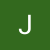# The Blind 75 Leetcode Series: Same TreePhoto by Chris Ried on Unsplash

Today, we are going to work on 100. Same Tree

Given the roots of two binary trees `p` and `q`, write a function to check if they are the same or not.

Two binary trees are considered the same if they are structurally identical, and the nodes have the same value.

We are given 2 trees, and each node has 2 children. We want to traverse through these trees to see if they are “the same”. The definition of “the same” here is when they have the same shape (ex. if node A is missing left branch, node A on the corresponding tree should have a missing left branch as well) and have the same values for corresponding nodes.

I don’t see corner case questions that would change how we proceed here, but it’s always nice to ask these questions to let your interviewers know that you don’t just jump into solving questions and that you think it through before proceeding.

So some questions could be

1. do we deal with empty tree?
2. do we expect the node values to go very large, like over int32 large?

If the answers are yes and no, then we can go ahead and start.

In order to check if 2 trees are the same, we want to go into node level and make sure both nodes at the same level are the same. There are 3 conditions we want to check to make sure a node is the same:

1. Do they both have left branch?
2. Do they both have right branch?
3. Do they have the same value?

Translating these into if-conditions, apply to every node, then traverse down to the children until we’ve hit all nodes, then we should know our answer.

If we reach the end for both trees and we still haven’t violated any of the conditions, they we return true. To traverse, we can simply call the same function (thus becoming a recursive) on both left children of the 2 trees and both right children of the 2 tress.

To put it into code, it looks like

`def isSameTree(p, q):    # we've reached the leaf node, return true    if not p and not q:        return True    # 2nd tree missing node    if not p and q:        return False    # 1st tree missing node    if not q and p:        return False    # 2 nodes' values don't match    if p.val != q.val:        return False    # traverse by checking the left children and right children    return isSameTree(p.left, q.left) and isSameTree(p.right, q.right)`

What’s the time complexity here? We want to traverse through the entire tree twice. If a tree has N nodes, then we are looking at O(2N) which becomes O(N) for time complexity.

Note that this recursion approach is using DFS as we keep checking the children first before returning the values to the upper level (`isSameTree(lefts) && isSameTree(rights)`). Can we get the same answers by using a BFS approach?

In order to achieve BFS, we need a stack or queue where we store the upcoming nodes in the queue and keep popping them until we have no more node in the queue. Every time we check a new node, we potentially store 2 more nodes in the queue. Since we pop from the beginning of the queue (if we are popping from the end of the queue, we are checking the children first again, thus making it a DFS), we always start with upper level, and we only advance to lower levels (towards the leaf nodes) when we finish the upper level.

We need a queue. A list CAN do the job, but since we are popping from the beginning of the queue, simply using `list.pop(0)` is actually an O(N) operation, which is not desirable. Instead, we use the `deque`. Again, deque stands for double-end queue. It makes `pop(0)` (which is called` popleft()`) an O(1) operation.

Putting it into code:

`from collections import dequedef isSameTree(p, q):    queue = deque()    # store the roots    queue.append((p, q))    while queue:        p, q = queue.popleft()        # we've reached the leaf node, but instead of returning true, we just keep going since we might still have nodes in queue        if not p and not q:            continue        if not p and q:            return False        if p and not q:            return False        if p.val != q.val:            return False        # add the children into the queue        queue.append((p.left, q.left))        queue.append((p.right, q.right))    return True`

The time complexity is still O(N) as we still need to traverse through the entire trees.

As we do more tree problems, we will see that DFS and BFS are constantly going to show up. Interviewers love this type of questions because they test the candidates the ability of knowing what the tree and queue would look like at any given time. I’ve even seen a problem which the interviewer already codes the solution out, and he would point at a random node and ask you what the queue looks like at this point. To me, this is actually a better problem compare to these leetcode problems, but this requires the interviewers to put in more thoughts and time into creating the problems, which a lot of people don’t want to do.

I’d recommend practicing with these problems, too, to see if you truly understand the concept.

That’s it! Another problem down.